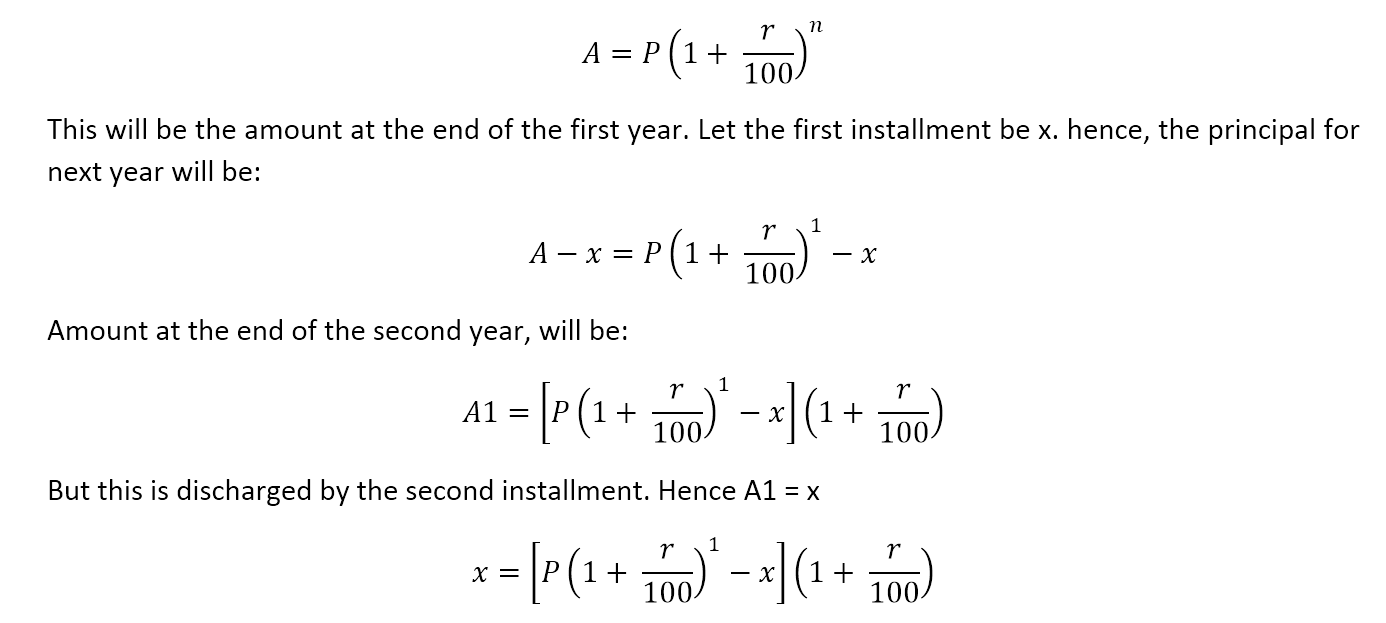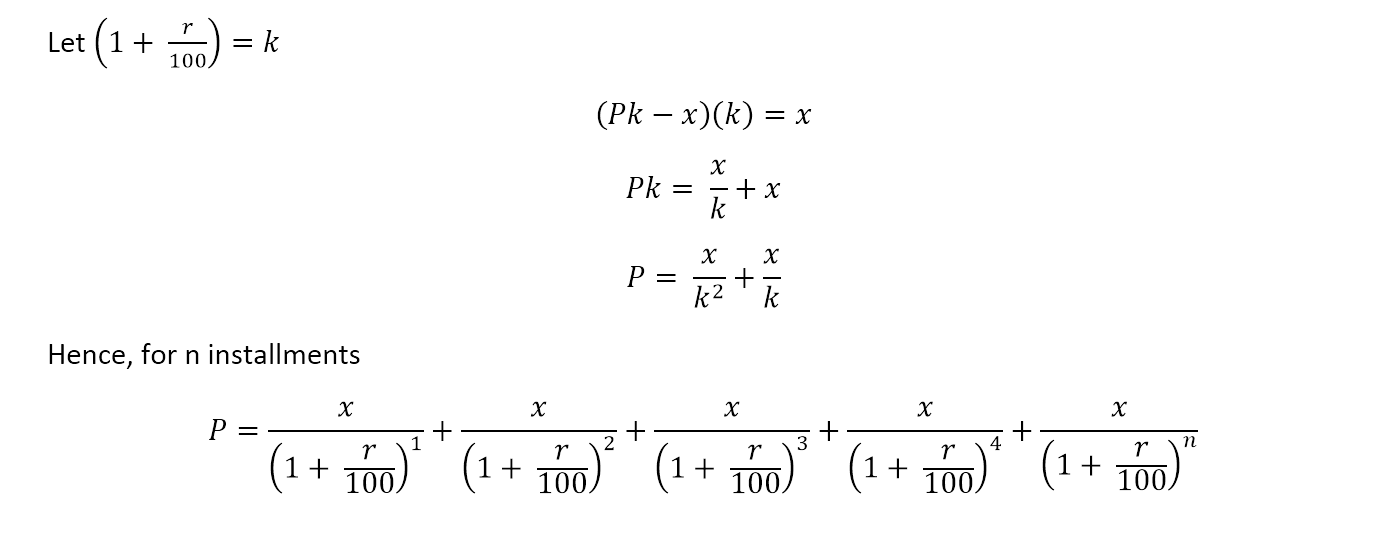Select Page

In the last article on Simple and Compound Interest, we looked at the basic concepts of simple interest and compound interest. We will continue this topic and understand the concept of installments.

What’s an installment? It is a sum of money due as one of several payments for something, spread over an agreed period of time. So if you buy a TV and you don’t have enough money to pay for it completely, you may agree on giving a certain money at the end of every month which will over a period of time compensate for your choice of not paying it at one go. Yes, you will end up paying more money than a one-time payment but you are getting the convenience of paying it over a period of time. So how to calculate installments?

Installment calculation can happen both in case of Simple Interest and Compound Interest. We will look at Installments in case of compound interest first.

Q. A person borrowed a sum P at rate of interest r, compounded annually. If the money is to be repaid in two equal annual installments, each payable at the end of the year, then what is the value of each installment?

Let us derive a formula to calculate this. We know thatBoost your CAT 2016 preparation by joining this group (for serious aspirants only)

And this is how we can calculate installments by using a simple formula.

Try this question: Three equal installments of 1000 Rs each discharged a sum borrowed at 20% compound interest compounded annually. Find the sum.

Simple substitution in our formula will give us: P=1000/(1+ 20/100)^1 + 1000/(1+ 20/100)^2 + 1000/(1+ 20/100)^3

P = 2106.48

We derived the entire equation to highlight the point that in case of compound interest, Principal at the end of first year (after paying the installment) = Principal + Interest for the first year – Installment. This is where compound interest is different from simple interest calculation. Let us look at Installments at Simple Interest now.

Q. A person borrowed a sum P at rate of simple interest r. If the money is to be repaid in two equal annual installments, each payable at the end of the year, then what is the value of each installment?

Now, in case of simple interest, only the installment is subtracted from the principal at the end of every year to get the principal for the calculation of next year’s interest and installment.

 Year Installment Principal Interest 1 x P Pr/100 2 x P-x (P-x)r/100 Total 2x Pr/100 + (P-x)r/100

And this will give us: 2x=P + Pr/100 + (P-x)r/100

If instead of two years, the question was for three years, the table would have appeared like this:

 Year Installment Principal Interest 1 x P Pr/100 2 x P-x (P-x)r/100 3 x P-2x (P-2x)r/100 Total 3x Pr/100 + (P-x)r/100 + (P-2x)r/100

And this will give us: 3x = P + Pr/100 + (P-x)r/100 + (P-2x)r/100

Try this question: Prasad borrowed 5000 Rs at 10% simple interest repayable in three equal installments. Find the installment.

Using our formula, for three equal installments, we get 3x = 5000 + (5000(10))/100 + (5000-x)10/100 + (5000-2x)10/100

On simplifying this, x = 1969.69

So the important point that one must understand is the way Principal is calculated at the end of every year in case of simple interest and compound interest. Hope you are finding our 75 days to CAT articles series useful. Any feedback and queries, drop a line in the comments section.

Boost your CAT 2016 preparation by joining this group (for serious aspirants only)

P.S.: We have explained this concept and many more such concepts in our awesome video course. Check it out here – CAT Complete Video Course

error: Content is protected !!